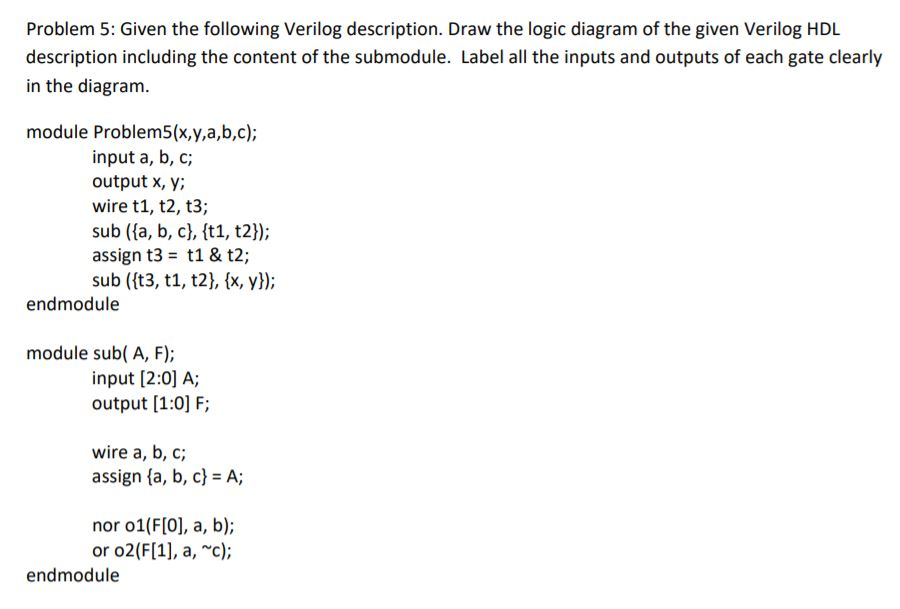# Question Problem 5: Given the following Verilog description. Draw the logic diagram of the given Verilog HDL description including the content of the submodule. Label all the inputs and outputs of each gate clearly in the diagram. module Problem5(x,y,a,b,c); input a, b, c; output x, y; wire t1, t2, t3; sub ({a,b,c}, {t1, t2}); assign t3 = t1 & t2; sub ({t3, t1, t2}, {x,y}); endmodule module sub( A, F); input (2:0) A; output (1:0) F; wire a, b, c; assign {a,b,c} = A; nor 01(F, a, b); or 02(F, a, c); endmoduleSRU6ZG The Asker · Electrical EngineeringTranscribed Image Text: Problem 5: Given the following Verilog description. Draw the logic diagram of the given Verilog HDL description including the content of the submodule. Label all the inputs and outputs of each gate clearly in the diagram. module Problem5(x,y,a,b,c); input a, b, c; output x, y; wire t1, t2, t3; sub ({a,b,c}, {t1, t2}); assign t3 = t1 & t2; sub ({t3, t1, t2}, {x,y}); endmodule module sub( A, F); input (2:0) A; output (1:0) F; wire a, b, c; assign {a,b,c} = A; nor 01(F, a, b); or 02(F, a, c); endmodule
More
Transcribed Image Text: Problem 5: Given the following Verilog description. Draw the logic diagram of the given Verilog HDL description including the content of the submodule. Label all the inputs and outputs of each gate clearly in the diagram. module Problem5(x,y,a,b,c); input a, b, c; output x, y; wire t1, t2, t3; sub ({a,b,c}, {t1, t2}); assign t3 = t1 & t2; sub ({t3, t1, t2}, {x,y}); endmodule module sub( A, F); input (2:0) A; output (1:0) F; wire a, b, c; assign {a,b,c} = A; nor 01(F, a, b); or 02(F, a, c); endmodule